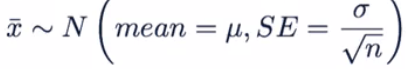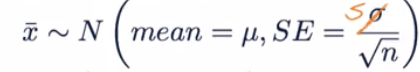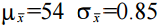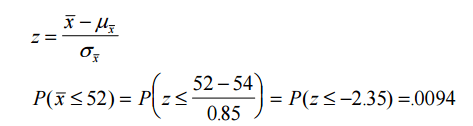### Central Limit Theorem

#### Central Limit Theorem (CLT):

The distribution of sample statistics is nearly normal, centered at the population mean, and with a standard deviation equal to the population standard deviation divided by square root of the sample size.

We can express the above statement using below notation.The above notation tells us three things.

1. The shape of the distribution of sample statistics is nearly normal. It means if we plot the sample statistics in a histogram we will get a bell shape curve.
2. The center of the sampling distribution will equal to population mean.
3. And the spread of the sampling distribution which we measured by standard error will be equal to standard deviation of population divided by square root of the sample size. Sometimes we don’t know about population standard deviation sigma or often we don’t have access to the whole population then we calculate standard deviation of sampling distribution which is also called standard error by using sample standard deviation S.#### Conditions for the CLT:

###### 1. Independence:
• Sampled observations must be independent.
• Random sample/random assignment
• If sampling without replacement, then needs to be n < 10% of population.

If we grab a very big portion of sample from the population sometimes it very difficult to make sure that sample individuals are independent of each other. Suppose you are doing some research on genetic applications and out of 10,000 people you surveyed 5000 from your city. Then it’s very difficult to make sure that your data in not genetically independent. Because you included 50% of the total population. Though we like large sample but we want to keep that limit somewhat proportional to our population and a good rule of thub is usually if we do sampling without replacement then n should not be more than 10% of the population.

###### 2. Sample size/ skew:
• Either the population distribution is normal, or if the population distribution is skewed, the sample size is large (rule of thumb: n > 30)

#### Explanation of CLT:

Frequently we interested in μ and estimate it using, so we need to know about the sampling distribution.

Theory says that for random samples of size n from any populationMu-X-bar is equal to Mu. Mu stands for the population mean, and Mu-X-bar stands for the mean of the sampling distribution of all the sample mean. The standard deviation of the sampling distribution is symbolized by Sigma-X-bar and is equal to Sigma divided by the square root of n. The X-bar is added to make clear that we are talking about the standard deviation of the sampling distribution in which the scores are sample means, or in other words, X-bars. Sigma stands for the standard deviation in the population. And n stands for the sample size.

According to CLT,  if n is sufficiently large (≥30) the sampling distribution ofwill also be approximately normal.

If you draw an infinite number of samples from a bell-shaped population distribution, the distribution of means from this infinite number of samples will be bell-shaped, and the mean of this distribution of sample means will be exactly the same as the population mean. We call this distribution the sampling distribution of the sample mean.

So, the central limit theorem says that, provided that the sample size is sufficiently large, the sampling distribution of sample mean X-bar has an approximately normal distribution. Even if the variable of interest is not normally distributed in the population! Isn’t that cool? No matter how a variable is distributed in the population, the sampling distribution of the sample mean is always approximately normal, as long as the sample size is large enough. As a guideline for ‘large enough’ a sample size of 30 or larger is often used as we discussed before.

##### Example of the Central Limit Theorem in Practice:

Roll 30 dice and calculate the average (sample mean) of the numbers that you get on each die. Now repeat this experiment 1000 times each time rolling 30 dice and computing a new sample mean. Plot a histogram of the 1000 sample means that you have obtained. This plot will look approximately normal.

##### Example 2:

Manufacturer claims the life of a battery type has a mean of 54 months and std dev of 6 months. A consumer group purchases a sample of 50 of these batteries and tests them. They find an average life of 52 months, what should they conclude?

If manufacturer’s claim is truen>30 so use central limit theorem.If the manufacturer’s claim is true then what the consumer group observed is very unlikely – a more plausible explanation is that the true value of μ is less than 54.